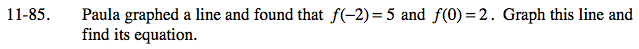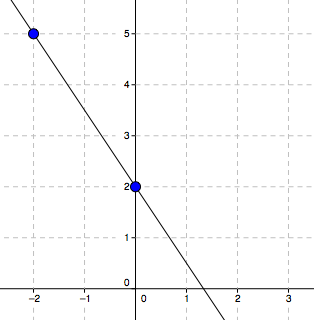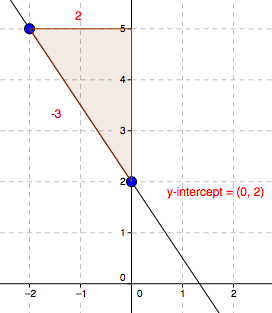### Home > AC > Chapter 11 > Lesson 11.2.1 > Problem11-85

11-85.f(−2) = 5 means (−2,5) is a point on the line. Also, (0,2) is a point on the line.

Graph the points on a set of axis, then draw the line.

To find the equation, make a slope triangle to find the slope and the y-intercept. Substitute these values into the equation of a line.